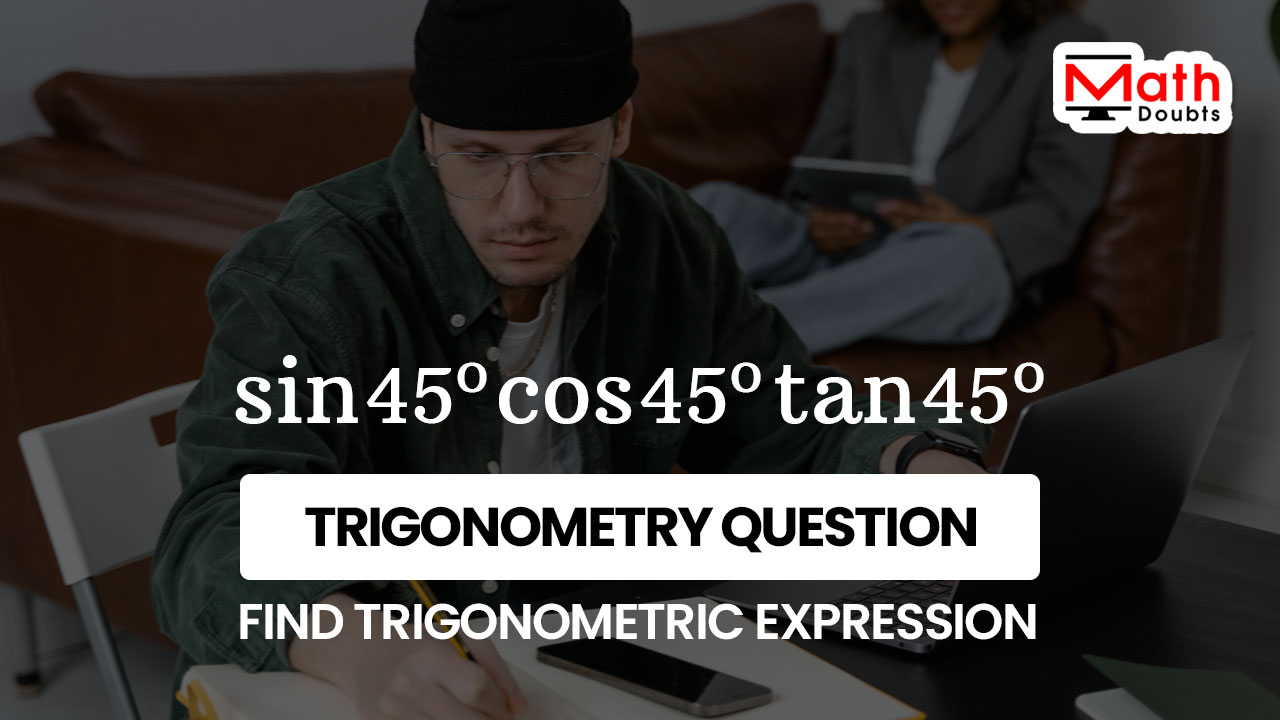# Evaluate $\sin{45^\circ}\cos{45^\circ}\tan{45^\circ}$In this trigonometry problem, sine, cosine and tangent are involved in multiplication to express their product in mathematical form when the angle of a right triangle is forty five degrees. So, the trigonometric expression that represents the product of the sine, cosine and tan of angle forty degrees should be evaluated by simplifying it.

### Express the Product in Multiplication form

The sine of angle forty five degrees, cosine of angle forty five degrees and tangent of angle forty five degrees are written in product form in the given trigonometric expression. So, let us expand the trigonometric expression firstly in multiplication form to start the process of simplifying the trigonometric expression.

$=\,\,\,$ $\sin{45^\circ}$ $\times$ $\cos{45^\circ}$ $\times$ $\tan{45^\circ}$

### Substitute the values of Trigonometric ratios

According to trigonometry, the sin 45 degrees value is equal to $1$ by squre root of $2$, the cos 45 degrees value is also equal to $1$ divided by $\sqrt{2}$ and the tan 45 degrees value is equal to $1$. Now, replace the values of them in the given trigonometric expression.

$=\,\,\,$ $\dfrac{1}{\sqrt{2}}$ $\times$ $\dfrac{1}{\sqrt{2}}$ $\times$ $1$

### Find the Arithmetic expression by simplification

Now, the trigonometric expression is converted as an arithmetic expression. It is time to simplify the arithmetic expression to find the value of the product of $\sin{45^\circ}$, $\cos{45^\circ}$ and $\tan{45^\circ}$.

Firstly, multiply the first two factors as per the multiplication rule of fractions.

$=\,\,\,$ $\dfrac{1 \times 1}{\sqrt{2} \times \sqrt{2}} \times 1$

$=\,\,\,$ $\dfrac{1}{\big(\sqrt{2}\big)^2} \times 1$

The multiplication of two irrational numbers in rational form formed a rational number as their product.

$=\,\,\,$ $\dfrac{1}{2} \times 1$

Now, multiply the rational number $1$ divided by $2$ by the integer $1$ as per the product rule of the rational numbers.

$=\,\,\,$ $\dfrac{1}{2}$

The rational number $1$ divided by $2$ can also be evaluated by the division method and its quotient is equal to $0.5$.

$=\,\,\,$ $0.5$

Latest Math Topics
Jun 26, 2023
Jun 23, 2023

###### Math Questions

The math problems with solutions to learn how to solve a problem.

Learn solutions

Practice now

###### Math Videos

The math videos tutorials with visual graphics to learn every concept.

Watch now

###### Subscribe us

Get the latest math updates from the Math Doubts by subscribing us.